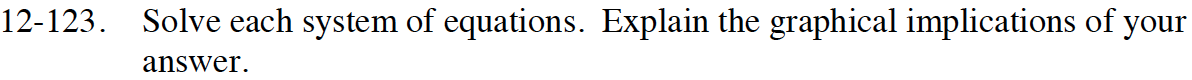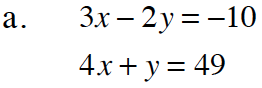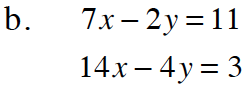Home > CCA2 > Chapter 12 > Lesson 12.2.2 > Problem12-123

12-123.
1. Solve each system of equations. Explain the graphical implications of your answer. Homework Help ✎

1. 3x − 2y = −10
4x + y = 49

2. 7x − 2y = 11
14x − 4y = 3Use the Elimination Method.

The two lines intersect at (8, 17).Use the same method as part (a).
Do you get an answer?
What does that answer tell you about the graph?